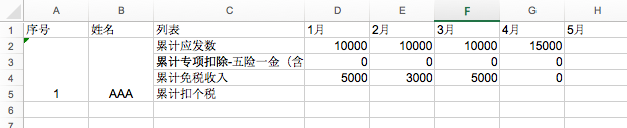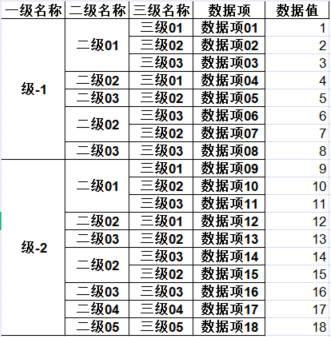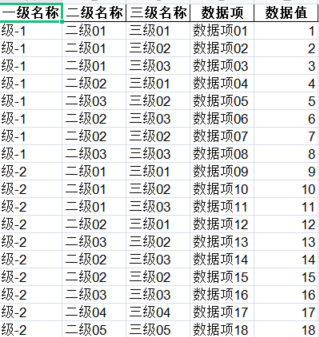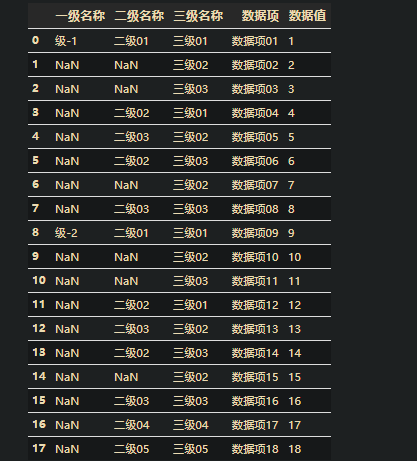• 读取 可以使用xlrd，也可以...xlrd也遇到了一点问题，文件如果是xls文件直接打开，在提取合并单元格的时候会拿不到数据，需要加上 formatting_info=True，formatting_info=True时候打开.xlsx文件会报错NotImple...
读取
可以使用xlrd，也可以使用openpyxl，但是openpyxl读取不了.xls格式的Excel，需要转成xlsx，有点麻烦，所以使用了xlrd。

打开文件
xlrd也遇到了一点问题，文件如果是xls文件直接打开，在提取合并单元格的时候会拿不到数据，需要加上 formatting_info=True，formatting_info=True的时候打开.xlsx文件会报错NotImplementedError: formatting_info=True not yet implemented，加个if就好了
workbook = xlrd.open_workbook(path)
if path.split('.xl') == 's':
workbook = xlrd.open_workbook(path, formatting_info=True)
# 获取sheet
sheet = workbook.sheet_by_index(0)


获取所有的合并单元格坐标# 获取行数
r_num = sheet.nrows
# 获取列数
c_num = sheet.ncols
merge = sheet.merged_cells
print(merge)   # [(1, 5, 0, 1), (1, 5, 1, 2)], 对应上面两个合并的单元格


组装数据
read_data =[]
for r in range(r_num):
li = []
for c in range(c_num):
# 读取每个单元格里的数据，合并单元格只有单元格内的第一行第一列有数据，其余空间都为空
cell_value = sheet.row_values(r)[c]
# 判断空数据是否在合并单元格的坐标中，如果在就把数据填充进去
if cell_value is None or cell_value == '':
for (rlow, rhigh, clow, chigh) in merge:
if rlow <= r < rhigh:
if clow <= c < chigh:
cell_value = sheet.cell_value(rlow, clow)
li.append(cell_value)
read_data.append(li)


写入
使用的是xlwt

直接上代码f = xlwt.Workbook()
sheet1 = f.add_sheet('sheet1', cell_overwrite_ok=True)
headers = ['序号', "姓名", '列表', '1月', '2月', '3月', '4月', '5月']
name = ['1-AAA', '2-BBB', '3-CCC', '4-DDD']
status = ['累计应发数', '累计专项扣除-五险一金', '累计免税收入', '累计扣个税']
# 生成第一行header
for i in range(0,len(headers)):
sheet1.write(0,i,headers[i])

# 生成序号、姓名单元格
i, j = 1, 0
while i < 10*len(name) and j < len(name):
sheet1.write_merge(i,i+9,0,0,name[j].split('-'))
sheet1.write_merge(i,i+9,1,1,name[j].split('-'))
i += 10
j += 1

#生成列表
i = 0
while i < 10*len(column0):
for j in range(0,len(status)):
sheet1.write(j+i+1,3,status[j])
i += 10
# 保存
f.save(path)


Django导出

服务端代码excel_stream = io.BytesIO()
# BytesIO流（在内存中读写）
f.save(excel_stream)
res = excel_stream.getvalue()
excel_stream.close()
response = HttpResponse(content_type='application/vnd.ms-excel')
from urllib import parse
file_name = str(path.name).split('.xls') + '(计算后).xls'
response['Content-Disposition'] = 'attachment;filename=' + parse.quote(file_name)
response.write(res)
return response


前端
写一个简单的form表单就行了

本人链接：时光不写博客


展开全文• 目录一、问题需求二、解决办法 一、问题需求      ...但是当程序需要分析或数据库使用时，就需要对这些合并的单元格进行拆分，并填补对应单元格中的数据。 想要的结果如
目录一、问题需求二、解决办法

一、问题需求
本篇博文可以说是博主另一篇博文的反向需求——博文：《python实现excel单元格对象合并》。
简单描述下问题，借助示例来说明，日常工作中，经常会拿到一些单元格合并的数据表，以下图为例。但是当程序需要分析或数据库使用时，就需要对这些合并的单元格进行拆分，并填补对应单元格中的数据。想要的结果如下图：二、解决办法
针对上文的问题描述，和想要的结果示例，使用python来实现这一问题的解决，可以说很方便。其实就是对相应的单元格进行填补。因为合并的单元格，python加载文件数据后，就只有对应单元格最上方的格子有值，其余的格子就是空的。加载后的结果如下：相应的解决方案就有了，就是对为空的单元格，采用向后填补的方法，就可以实现。对应的代码如下：
import pandas as pd

data = pd.read_excel(r'C:/Users/14369/Desktop/博文markdown版/测试数据结果.xls')
data.columns

data.fillna(method='ffill',inplace=True)
data.to_excel(r'C:/Users/14369/Desktop/博文markdown版/测试数据结果2.xls',index=False)


结果图就是问题需求描述中的结果示例。

扫码关注下方博主的微信公众号，回复 关键字  【单元格】,获得博文中提到的测试数据及结果，一起见证奇迹。展开全文• python 读取excel合并单元格 并将合并单元格的数据填充 代码如下： #找出合并单元格的数据 full_file_path指传入表格路径  def enter(self,full_file_path):  try:  data = xlrd.open_workbook(full_...
python 读取excel合并单元格  并将合并单元格的数据填充    代码如下：
#找出合并单元格的数据  full_file_path指传入的表格的路径
def enter(self,full_file_path):
try:
data = xlrd.open_workbook(full_file_path);
table = data.sheets()
'''获取合并单元格的数据[(0,5,0,1),(6,10,0,1)]  格式为列表下多个元组
一个元组代表一个起合并单元格
(a,b,c,d)    a为起始行 b为结束行  c为起始列 d为结束列  左闭右开原则'''

lists=table.merged_cells;
except Exception as ex:
print(ex)
mergeJson={};   #合并单元格与该单元格的数据
nrows = table.nrows #行数
#找出合并单元格  并找出合并单元格的值  以键值的方式存储   元组为键   该合并单元格的值为值
for i in range(1,nrows):
rowValues= table.row_values(i) #某一行数据
event = {}
for j in range(0,len(rowValues)):  #某一行中列的数据
for mer in lists:   #遍历合并单元格的列表   mer为元组
if i>=mer and i<mer and j>=mer and j<mer :  #判断是否是合并行
#处理合并行
if rowValues[j] != "" :    #不为空的合并值
mergeJson[mer]=rowValues[j];

''' 将合并的单元格和数据传过来  处理数据  此处的处理逻辑是 将合并的单元格的位置确定 判断读取的当前单元格在不在合并单元格内 在的话 就把为空的单元格的值填充为合并单元格的值'''
def dealData(self,data,mergeJson)：
bulk = []
table = data.sheets()
lists=table.merged_cells;
nrows = table.nrows #行数
#解析数据
try :
for i in range(1,nrows):
rowValues= table.row_values(i) #某一行数据
event = {}
for j in range(0,len(rowValues)):  #某一行中列的数据
if mergeJson:
for tuplerc in mergeJson:   #data为字典  key 为元组   value  为该合并单元格的值
if i>=tuplerc and i<tuplerc and j>=tuplerc and j<tuplerc :  #判断是否是合并行
#处理合并行
if rowValues[j] == "" :    #不为空的合并值
rowValues[j]= mergeJson[tuplerc];
else :
event[fields_names[j]]=value;
else :
event[fields_names[j]] =rowValues[j]
bulk.append(event)
except Exception as ex:
print(ex)
print (bulk);


展开全文• ![图片说明](https://img-ask.csdn.net/upload/202008/13/1597317945_734276.png) !... 利用docx处理word中表格，在处理合并单元格报错。 但是，在删除图2中红色框内的单元格内容后，可以正常运行。
• 最近在工作中需要批量处理一些Excel文件，为了更直观的展示数据，需要对Excel中的单元格进行合并处理。因为一直使用Pandas实现Excel读写操作，而Pandas无法将单元格进行合并，利用Python的xlsxwriter模块可以实现...
利用Python实现Excel合并单元格
最近在工作中需要批量处理一些Excel文件，为了更直观的展示数据，需要对Excel中的单元格进行合并处理。因为一直使用Pandas实现Excel读写操作，而Pandas无法将单元格进行合并，利用Python的xlsxwriter模块可以实现Excel合并单元格。帖子 python之DataFrame写excel合并单元格 中提供了一种方法，但在使用中会出现一些问题，比如无法处理字符串等类型数据。通过修改方法，定义了一个excel_merge_cells函数，基本可以满足日常利用Python实现Excel合并单元格的需求。
原帖方法
在工作中经常遇到需要将数据输出到excel，且需要对其中一些单元格进行合并，比如如下表表格，需要根据A列的值，合并B、C列的对应单元格1、定义一个MY_DataFrame类，继承DataFrame类，这样能很好的利用pandas的很多特性，而不用自己重新组织数据结构。
2、定义一个my_mergewr_excel方法，参数分别为：输出excel的路径、用于判断是否需要合并的key_cols列表、用于指明哪些列上的单元格需要被合并的列表
3、将MY_DataFrame封装为一个My_Module模块，以备重用。
合并的算法如下：
1、根据给定参数的【关键列】，进行分组计数和排序，添加CN和RN两个辅助列
2、判断CN大于1的，该分组需要合并，否则该分组（行）无需合并（CN=1说明这个分组数据行是唯一的，无需合并）
3、对应需要合并的分组，判断当前列是不是在给定参数【合并列】中，是则用合并写excel单元格，否则就是普通的写excel单元格。
4、在需要合并的列中，如果对于的RN=1则调用merge_range，一次性写想下写CN个单元格，如果RN>1则跳过该单元格，因为在RN=1的时候，已经合并写了该单元格，若再重复调用erge_range，打开excel文档时会报错。
用图解释如下：具体代码如下：

# -*- coding: utf-8 -*-
"""
Created on 20170301
@author: ARK-Z
"""
import xlsxwriter
import pandas as pd

class My_DataFrame(pd.DataFrame):
def __init__(self, data=None, index=None, columns=None, dtype=None, copy=False):
pd.DataFrame.__init__(self, data, index, columns, dtype, copy)

def my_mergewr_excel(self,path,key_cols=[],merge_cols=[]):
# sheet_name='Sheet1', na_rep='', float_format=None, columns=None, header=True, index=True, index_label=None, startrow=0, startcol=0, engine=None, merge_cells=True, encoding=None, inf_rep='inf', verbose=True):
self_copy=My_DataFrame(self,copy=True)
line_cn=self_copy.index.size
cols=list(self_copy.columns.values)
if all([v in cols for i,v in enumerate(key_cols)])==False:     #校验key_cols中各元素 是否都包含与对象的列
print("key_cols is not completely include object's columns")
return False
if all([v in cols for i,v in enumerate(merge_cols)])==False:  #校验merge_cols中各元素 是否都包含与对象的列
print("merge_cols is not completely include object's columns")
return False

wb2007 = xlsxwriter.Workbook(path)
worksheet2007 = wb2007.add_worksheet()
format_top = wb2007.add_format({'border':1,'bold':True,'text_wrap':True})
format_other = wb2007.add_format({'border':1,'valign':'vcenter'})
for i,value in enumerate(cols):  #写表头
#print(value)
worksheet2007.write(0,i,value,format_top)

#merge_cols=['B','A','C']
#key_cols=['A','B']
if key_cols ==[]:   #如果key_cols 参数不传值，则无需合并
self_copy['RN']=1
self_copy['CN']=1
else:
self_copy['RN']=self_copy.groupby(key_cols,as_index=False).rank(method='first').ix[:,0] #以key_cols作为是否合并的依据
self_copy['CN']=self_copy.groupby(key_cols,as_index=False).rank(method='max').ix[:,0]
#print(self)
for i in range(line_cn):
if self_copy.ix[i,'CN']>1:
#print('该行有需要合并的单元格')
for j,col in enumerate(cols):
#print(self_copy.ix[i,col])
if col in (merge_cols):   #哪些列需要合并
if self_copy.ix[i,'RN']==1:  #合并写第一个单元格，下一个第一个将不再写
worksheet2007.merge_range(i+1,j,i+int(self_copy.ix[i,'CN']),j, self_copy.ix[i,col],format_other) ##合并单元格，根据LINE_SET判断需要合并几个
#worksheet2007.write(i+1,j,df.ix[i,col])
else:
pass
#worksheet2007.write(i+1,j,df.ix[i,j])
else:
worksheet2007.write(i+1,j,self_copy.ix[i,col],format_other)
#print(',')
else:
#print('该行无需要合并的单元格')
for j,col in enumerate(cols):
#print(df.ix[i,col])
worksheet2007.write(i+1,j,self_copy.ix[i,col],format_other)

wb2007.close()
self_copy.drop('CN', axis=1)
self_copy.drop('RN', axis=1)


调用代码：


import My_Module

DF=My_DataFrame({'A':[1,2,2,2,3,3],'B':[1,1,1,1,1,1],'C':[1,1,1,1,1,1],'D':[1,1,1,1,1,1]})

DF
Out:
A  B  C  D
0  1  1  1  1
1  2  1  1  1
2  2  1  1  1
3  2  1  1  1
4  3  1  1  1
5  3  1  1  1

DF.my_mergewr_excel('000_2.xlsx',['A'],['B','C'])


————————————————
版权声明：本文为CSDN博主「周小科」的原创文章，遵循CC 4.0 BY-SA版权协议，转载请附上原文出处链接及本声明。
原文链接：https://blog.csdn.net/cakecc2008/article/details/59203980
修改后方法
利用Python进行Excel文件读取并分析多数采用Pandas模块进行，所以直接将方法定义为一个名为 excel_merge_cells 的函数，代码如下：
import pandas as pd
import xlsxwriter

def excel_merge_cells(df, save_name, key_cols=[], merge_cols=[]):
'''key_cols：用于判断是否需要合并的key_cols列表
merge_cols：用于指明哪些列上的单元格需要被合并的列表'''
self_copy = df.copy(deep=True)
line_cn = self_copy.shape
self_copy.index = list(range(line_cn))
cols = list(self_copy.columns)
self_copy['temp_col'] = 1
if all([v in cols for v in key_cols]) == False:   # 校验key_cols中各元素 是否都包含与对象的列
raise ValueError("key_cols is not completely include object's columns")
if all([v in cols for v in merge_cols]) == False: # 校验merge_cols中各元素 是否都包含与对象的列
raise ValueError("merge_cols is not completely include object's columns")

wb = xlsxwriter.Workbook(save_name)
worksheet = wb.add_worksheet()
format_top = wb.add_format({'border':1, 'bold':True, 'text_wrap':True})
format_other = wb.add_format({'border':1,'valign':'vcenter'})

for i, value in enumerate(cols):  # 写表头
worksheet.write(0, i, value, format_top)

if key_cols == []:       # 如果key_cols 参数不传值，则无需合并，RN和CN为辅助列
self_copy['CN'] = 1  # 判断CN大于1的，该分组需要合并，否则该分组（行）无需合并（CN=1说明这个分组数据行是唯一的，无需合并）
self_copy['RN'] = 1  # RN为需要合并一组中第几行，CN=1，RN=1；CN=5，RN=1,...5
else:
self_copy['CN'] = self_copy.groupby(key_cols, as_index=False)['temp_col'].rank(method='max')['temp_col']    # method='max'，对整个组使用最大排名
self_copy['RN'] = self_copy.groupby(key_cols, as_index=False)['temp_col'].rank(method='first')['temp_col']  # method='first'，按照值在数据中出现的次序分配排名

for i in range(line_cn):
if self_copy.loc[i, 'CN'] > 1:
for j, col in enumerate(cols):
if col in (merge_cols):
if self_copy.loc[i, 'RN'] == 1: # 合并写第一个单元格，下一个第一个将不再写
worksheet.merge_range(i+1, j, i+int(self_copy.loc[i, 'CN']), j, self_copy.loc[i, col], format_other)
'''合并 开始行，开始列，结束行，结束列，值，格式'''
'''因为已经写了表头所以从i+1行开始写'''
else:
pass
else:
worksheet.write(i+1, j, self_copy.loc[i, col], format_other)
else:
for j, col in enumerate(cols):
worksheet.write(i+1, j, self_copy.loc[i, col], format_other)

wb.close()

函数需要传入的参数如下：

df：需要进行合并单元格处理的DataFrame
save_name：处理结果需要保存的路径及文件名
key_cols：用于判断是否需要合并的key_cols列表
merge_cols：用于指明哪些列上的单元格需要被合并的列表


展开全文excel 数据分析
• 客户这边，其中有一个如同上图所示 sheet，然而需求是，需要将这张表第一列（一级部）数据相同内容做合并单元格处理，结果如下： 代码分享如下： from openpyxl import load_workbook """合并单元格""" ...
• 主要介绍了Python基于xlrd模块处理合并单元格,文中通过示例代码介绍非常详细，对大家学习或者工作具有一定参考学习价值,需要朋友可以参考下
• python使用xlwt形成合并单元格的excel并且读取合并单元格的excel 在日常数据报表数据处理中，经常会遇到看某个大类下面每个小类各自情况，此时形成的合并一些单元格作为表头...1、安装python处理excel需要包...多级表头
• python read_excel读取文件，文件存在合并单元格，如何处理。 目前想法是将四行列名进行拼接，求指导，谢谢
• 处理excel表格时候经常遇到合并单元格的情况，使用xlrd中merged_cells方法可以获取当前文档中所有合并单元格的位置信息。 import xlrd xls = xlrd.open_workbook('test.xls') sh = xls.sheet_by_index(0) ...
• python docx模块读取word表 遇到合并单元格的处理 通过docx模块，读取word里面表格，并进行修改。 现在我遇到表格是这样： 里面行，列均有合并单元格的情况，为了正确读取到位置，用下面代码做了个试验，判断每...
• 这篇文章主要介绍了python操作openpyxl导出Excel 设置单元格格式及合并处理代码实例,文中通过示例代码介绍非常详细，对大家学习或者工作具有一定参考学习价值,需要朋友可以参考下贴上一个例子，里面设计很多...
• 前言：昨天获得一个数据处理的小需求，比较简单，用VBA也能做，不过最近Python比较多，所以用Python写了，效率也很快。 需求： 有一张总表，如下图所示 需要根据左侧工号和姓名，合并右侧获奖情况，结果如下...excel 数据分析
• 出现这种错误一般都是在用方法：ws.merge_cells() 合并单元格后，直接给单元格赋值导致。 经过我不断尝试，发现在合并单元格的初始位置赋值就不会出现问题。 比如以下代码： from openpyxl import Workbook wb =...excel
• 主要介绍了python操作openpyxl导出Excel 设置单元格格式及合并处理代码实例,文中通过示例代码介绍非常详细，对大家学习或者工作具有一定参考学习价值,需要朋友可以参考下
• 处理excel表格时候经常遇到合并单元格的情况，使用xlrd中merged_cells方法可以获取当前文档中所有合并单元格的位置信息。 import xlrd xls = xlrd.open_workbook('test.xls') sh = xls.sheet_by_index(0)...
• 接下来我们说一下excel单元格合并的方法，即将左表处理成右表形式 一、直接在excel文件上操作 1.wps点选 选择待合并区域，选择“开始” --> “合并居中” --> “合并相同单元格” 2.快捷方式 选择待合并区域...excel 数据分析
• python处理excel合并前言源码 前言 本篇博客是记录自己处理班级早起打卡，日常学习情况（数据来源与腾讯文档收集） 源码 import xlrd import xlwt import os """ xlrd操作： 1、常用单元格数据类型 ♦ 0. ...excel
• 1.第一列Sku数值相同合并A-E列，J-M列 2.M列设置行高完全显示内容 3.A1单元格内数字是SKU个数，合并单元格后数字更新 二、效果图 input入力文件处理前： 文件处理后 出力效果： 三、代码 1.合并...pandas openpyxl
• Python基于openpyxl模块对Excel合并单元格的处理： #!/usr/bin/env python # coding=utf-8 import openpyxl excelFile = 'test.xlsx' wb = openpyxl.load_workbook(excelFile) sheet = wb.sheetnames # 遍列Excel...excel
• 贴上一个例子，里面设计很多用法，根据将相同日期某些行合并处理。 from openpyxl import Workbook ...
• xlrd在读取合并单元格时，认为合并后的单元格的首格有值，其余格均为空，所以需要经过处理，在处理过程中考虑到假如又存在本身单元格内容就是空值的情况，所以需要计算合并的单元格的范围，在网上经过一番查找，发现...
• 如何提取word中的表格内容，对合并的行列内容能够准确提取，用python可以实现吗？ 插入链接与图片 链接: link. 图片: 带尺寸的图片:居中的图片: 居中并且带尺寸的图片: 当然，我们为了让用户更加便捷，...# python处理合并的单元格python 订阅